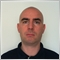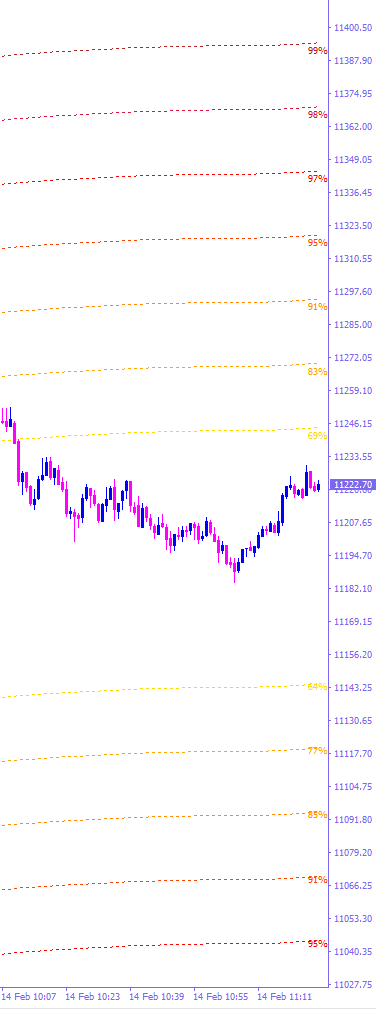# Help needed. How to update the indicator with each new bar?94

Hello everybody,

I'm a beginner in the programmation, and I have a question.
At each new bar, the text of my programm shifts a little more to the left, and I would like to keep it on the right side of the chart.

Unfortunately I didn't found the solution. I know that's is simple but...

Would someone have a suggestion to fix it?

(you can found the code below)

----------------------------------------------------------------------------------------------------

#property indicator_chart_window

int init(){
return(0);
}

int deinit(){
ObjectsDeleteAll();
return(0);
}

int start(){
//find index of bar shown at leftmost side of screen
int i = Bars-IndicatorCounted()-1;
if( i > 1 )
i = 0;

//work from leftmost bar to rightmost bar
while(i>=0){

//find current MA value, will also be used as y-coordinate
double currentMA = iMA(NULL,0,50,0,MODE_SMMA,PRICE_TYPICAL,i);
ObjectCreate( "maValue"+i,OBJ_TEXT,0,Time[i],currentMA+4900*Point);
ObjectSetText("maValue"+i,DoubleToStr("x",Digits-2)+"%",7,"Tahoma",Gold);

double currentMA1 = iMA(NULL,0,50,0,MODE_SMMA,PRICE_TYPICAL,i);
ObjectCreate( "maValue1"+i,OBJ_TEXT,0,Time[i],currentMA1+7400*Point);
ObjectSetText("maValue1"+i,DoubleToStr("x",Digits-2)+"%",7,"Tahoma",Orange);

double currentMA2 = iMA(NULL,0,50,0,MODE_SMMA,PRICE_TYPICAL,i);
ObjectCreate( "maValue2"+i,OBJ_TEXT,0,Time[i],currentMA2+9900*Point);
ObjectSetText("maValue2"+i,DoubleToStr("x",Digits-2)+"%",7,"Tahoma",DarkOrange);

double currentMA3 = iMA(NULL,0,50,0,MODE_SMMA,PRICE_TYPICAL,i);
ObjectCreate( "maValue3"+i,OBJ_TEXT,0,Time[i],currentMA3+12400*Point);
ObjectSetText("maValue3"+i,DoubleToStr("x",Digits-2)+"%",7,"Tahoma",OrangeRed);

double currentMA4 = iMA(NULL,0,50,0,MODE_SMMA,PRICE_TYPICAL,i);
ObjectCreate( "maValue4"+i,OBJ_TEXT,0,Time[i],currentMA4+14900*Point);
ObjectSetText("maValue4"+i,DoubleToStr("x",Digits-2)+"%",7,"Tahoma",Red);

double currentMA5 = iMA(NULL,0,50,0,MODE_SMMA,PRICE_TYPICAL,i);
ObjectCreate( "maValue5"+i,OBJ_TEXT,0,Time[i],currentMA5+17400*Point);
ObjectSetText("maValue5"+i,DoubleToStr("x",Digits-2)+"%",7,"Tahoma",Crimson);

double currentMA6 = iMA(NULL,0,50,0,MODE_SMMA,PRICE_TYPICAL,i);
ObjectCreate( "maValue6"+i,OBJ_TEXT,0,Time[i],currentMA6+19900*Point);
ObjectSetText("maValue6"+i,DoubleToStr("x",Digits-2)+"%",7,"Tahoma",FireBrick);

double currentMA7 = iMA(NULL,0,50,0,MODE_SMMA,PRICE_TYPICAL,i);
ObjectCreate( "maValue7"+i,OBJ_TEXT,0,Time[i],currentMA7-4900*Point);
ObjectSetText("maValue7"+i,DoubleToStr("x",Digits-2)+"%",7,"Tahoma",C'255,217,2');

double currentMA8 = iMA(NULL,0,50,0,MODE_SMMA,PRICE_TYPICAL,i);
ObjectCreate( "maValue8"+i,OBJ_TEXT,0,Time[i],currentMA8-7400*Point);
ObjectSetText("maValue8"+i,DoubleToStr("x",Digits-2)+"%",7,"Tahoma",Orange);

double currentMA9 = iMA(NULL,0,50,0,MODE_SMMA,PRICE_TYPICAL,i);
ObjectCreate( "maValue9"+i,OBJ_TEXT,0,Time[i],currentMA9-9900*Point);
ObjectSetText("maValue9"+i,DoubleToStr("x",Digits-2)+"%",7,"Tahoma",DarkOrange);

double currentMA10 = iMA(NULL,0,50,0,MODE_SMMA,PRICE_TYPICAL,i);
ObjectCreate( "maValue10"+i,OBJ_TEXT,0,Time[i],currentMA10-12400*Point);
ObjectSetText("maValue10"+i,DoubleToStr("x",Digits-2)+"%",7,"Tahoma",OrangeRed);

double currentMA11 = iMA(NULL,0,50,0,MODE_SMMA,PRICE_TYPICAL,i);
ObjectCreate( "maValue11"+i,OBJ_TEXT,0,Time[i],currentMA11-14900*Point);
ObjectSetText("maValue11"+i,DoubleToStr("x",Digits-2)+"%",7,"Tahoma",Red);

double currentMA12 = iMA(NULL,0,50,0,MODE_SMMA,PRICE_TYPICAL,i);
ObjectCreate( "maValue12"+i,OBJ_TEXT,0,Time[i],currentMA12-17400*Point);
ObjectSetText("maValue12"+i,DoubleToStr("x",Digits-2)+"%",7,"Tahoma",Crimson);

double currentMA13 = iMA(NULL,0,50,0,MODE_SMMA,PRICE_TYPICAL,i);
ObjectCreate( "maValue13"+i,OBJ_TEXT,0,Time[i],currentMA13-19900*Point);
ObjectSetText("maValue13"+i,DoubleToStr("x",Digits-2)+"%",7,"Tahoma",FireBrick);

i--;
}

return(0);
}

--------------------------------------------------------------------------------------------------------------

Thank you in advance for your help.369

I think the reason is that an object of the same name already exists. You try to create text labels named "maValue"+i, but on subsequent calls i will almost always be equal to 0. So you subsequently try to create labels named "maValue0", but such an object already exists. There's a return value for ObjectCreate. Check it. And just to be sure you don't get a name clash, try 'maValue_'+i.94

Hello Lippmaje,

Thanks for your suggestion and your interest.

I tried it, but at the end I obtained only 1 object in the screen, and it still moving at each new bar...

This programm is the 2nd part of a custom Indicator (see below)My problem is that the differents values (%) are Moving on the left side at each new bar.

I know that the solution is to put "datetime" or "newbar" somewhere...but I don't know where.

I tried many configurations, but without success.369

Use ObjectDelete to remove the labels from the previous run. Or just ObjectsDeleteAll(0,"maValue").94

Wonderful! Now it works correctly.

Thanks a lot for your help Lippmaje,

and God bless you.